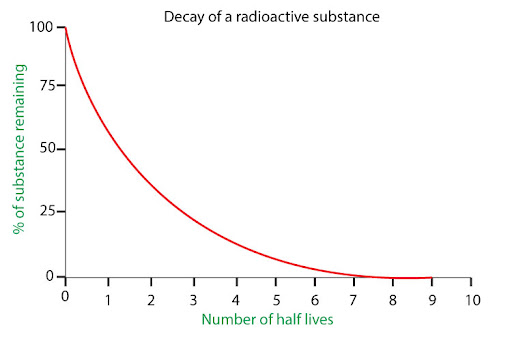# what is a half life of a substance and how can you work it out.

A: The half life of a radioactive substance is the time taken for a specific sample to decay by half. This is equivalent to the time taken for the same substance to have its decay rate drop to half its original value.

Experimentally, one would expect to find the half life in a pretty direct way: just measure the initial mass of the radioactive substance, wait until it weighs about half that amount, and then see how much time was spent. Simple as that.

But there’s a slight problem with that: while this method is sufficient for radioactive isotopes like Technetium-99 with a half life of 9 hours, it's not so good for elements like Uranium-238 with a half life of 4.5 billion years! So a better way to handle this is through some quick math.

We can find the number of unstable nuclei remaining after some time t using the equation:

N(t) = N(0) *e-λt
Where: N(t) =remaining amount of substance after time t
N(0) = initial amount of substance at t =0
t = time
λ= decay constant (probability of decay per unit time)
Now, we know that for an initial amount of substance N, after one half life T has passed (meaning t = T), the substance’s amount decreases by half.

At t = T (half life), N(T) = N/2

Therefore: N/2 = N * e-λT

1/2 = e-λT

2 = eλT

Taking the natural log on both sides:
ln(2) = ln(eλT )
ln(2) = T * ln(e)
ln(2) = T
﻿And now we rearrange:
T = ln(2)/λ
So, by knowing the decay constant (which can be found experimentally!), you can solve half life!